CBSE Class 12 Sample Paper for 2022 Boards (MCQ Based - for Term 1)

Class 12
Solutions of Sample Papers and Past Year Papers - for Class 12 Boards

## The points on the curve x 2 /9+y 2 /16 = 1 at which the tangents are parallel to y-axis are: (a) (0, ± 4)      (b) (±4, 0) (c) (±3, 0)       (d) (0, ±3)

This question is inspired from Ex 6.3,13 - Chapter 6 Class 12 - Application of Derivatives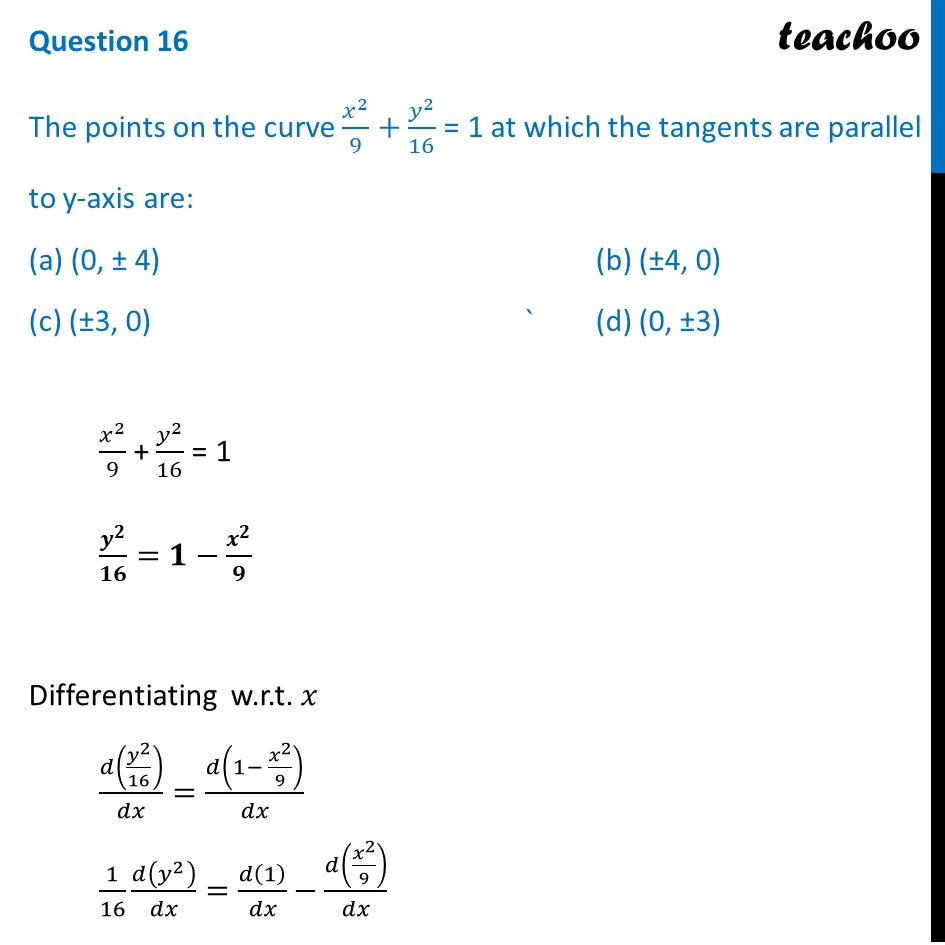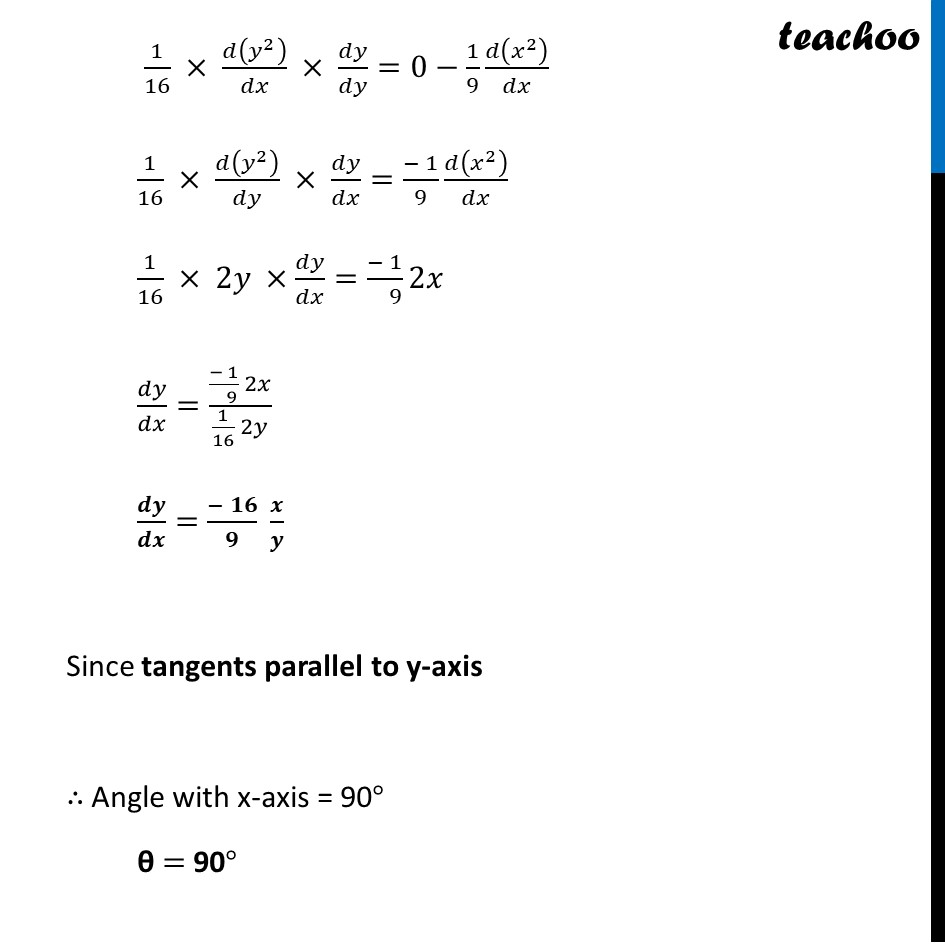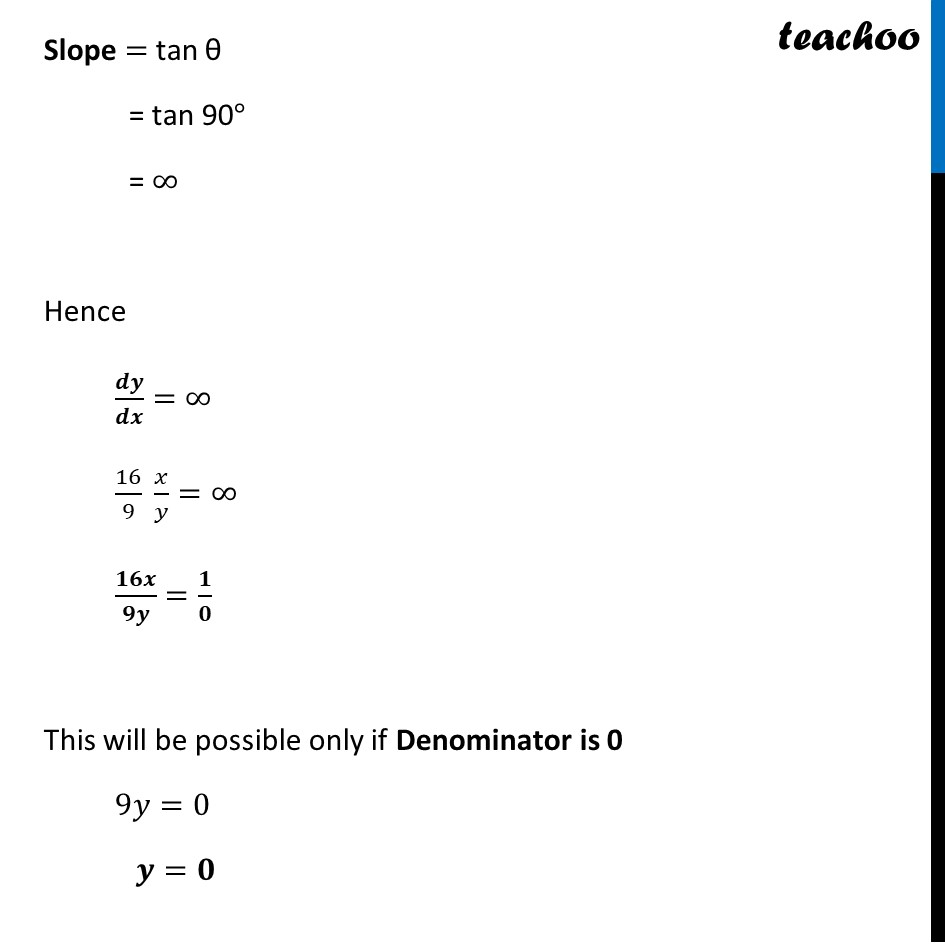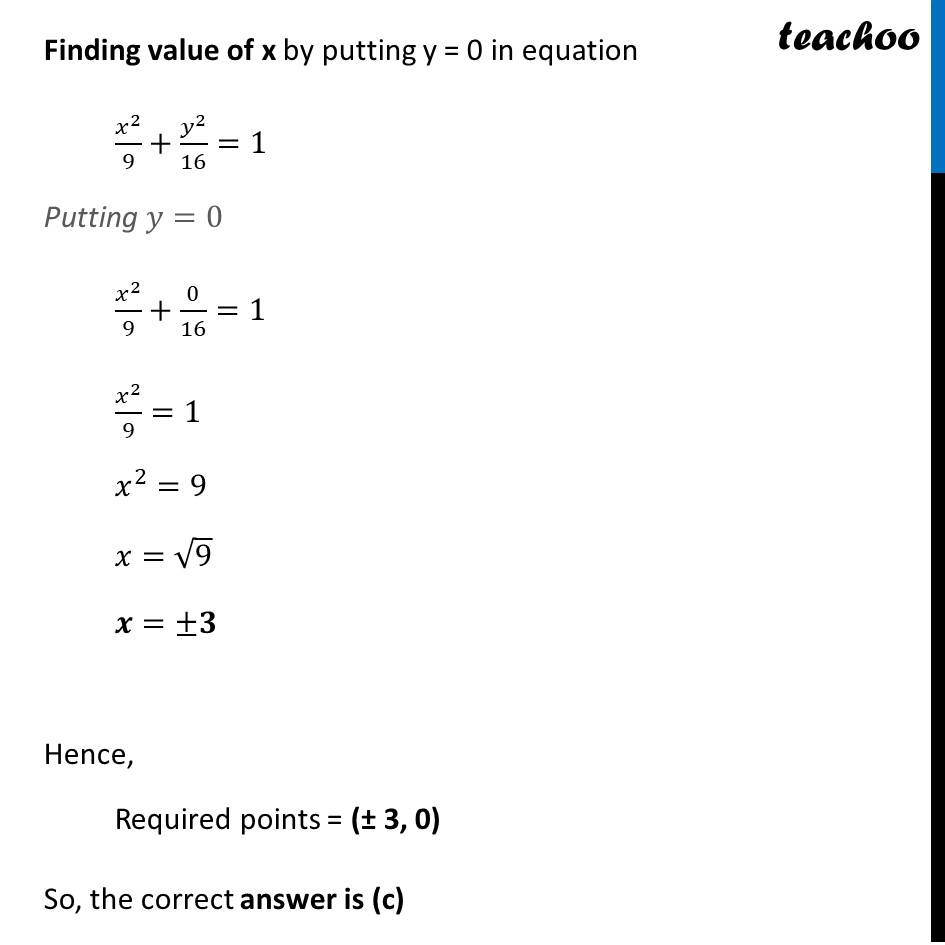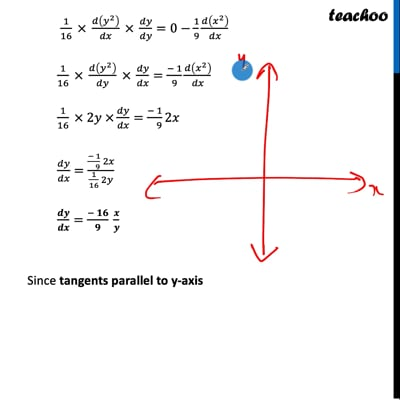This video is only available for Teachoo black users

Introducing your new favourite teacher - Teachoo Black, at only ₹83 per month

### Transcript

Question 16 The points on the curve 𝑥^2/9+𝑦^2/16 = 1 at which the tangents are parallel to y-axis are: (a) (0, ± 4) (b) (±4, 0) (c) (±3, 0) ` (d) (0, ±3) 𝑥^2/9 + 𝑦^2/16 = 1 𝒚^𝟐/𝟏𝟔=𝟏−𝒙^𝟐/𝟗 Differentiating w.r.t. 𝑥 𝑑(𝑦^2/16)/𝑑𝑥=𝑑(1− 𝑥^2/9)/𝑑𝑥 1/16 𝑑(𝑦^2 )/𝑑𝑥=𝑑(1)/𝑑𝑥−𝑑(𝑥^2/9)/𝑑𝑥 1/16 × 𝑑(𝑦^2 )/𝑑𝑥 × 𝑑𝑦/𝑑𝑦=0−1/9 𝑑(𝑥^2 )/𝑑𝑥 1/16 × 𝑑(𝑦^2 )/𝑑𝑦 × 𝑑𝑦/𝑑𝑥=(− 1)/9 𝑑(𝑥^2 )/𝑑𝑥 1/16 × 2𝑦 ×𝑑𝑦/𝑑𝑥=(− 1)/( 9) 2𝑥 𝑑𝑦/𝑑𝑥=((− 1)/( 9) 2𝑥)/(1/16 2𝑦) 𝒅𝒚/𝒅𝒙=(− 𝟏𝟔)/𝟗 𝒙/𝒚 Since tangents parallel to y-axis ∴ Angle with x-axis = 90° θ = 90° Slope = tan θ = tan 90° = ∞ Hence 𝒅𝒚/𝒅𝒙=∞ 16/9 𝑥/𝑦=∞ 𝟏𝟔𝒙/𝟗𝒚=𝟏/𝟎 This will be possible only if Denominator is 0 9𝑦=0 𝒚=𝟎 Finding value of x by putting y = 0 in equation 𝑥^2/9+𝑦^2/16=1 Putting 𝑦=0 𝑥^2/9+0/16=1 𝑥^2/9=1 𝑥^2=9 𝑥=√9 𝒙=±𝟑 Hence, Required points = (± 3, 0) So, the correct answer is (c)